# Grouped Frequency Tables

GCSELevel 6-7Cambridge iGCSE

## Grouped Frequency Tables

When there is a lot of data, it can be easier to separate the values into groups. We can then display the amount of data in each group in a grouped frequency table. We can also estimate the mean of a set of data from a grouped frequency table.

Make sure you are happy with the following topics before continuing:

## Grouped Frequency Tables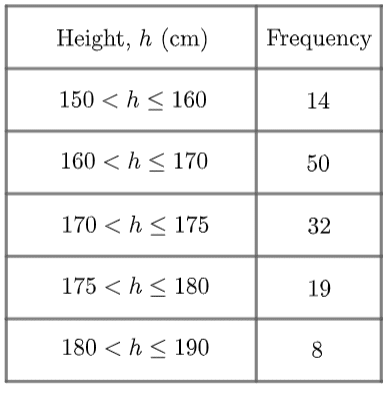This particular example is a set of data on the heights of a selection of adults. To understand what this table is saying, let’s look at the first group (also known as a class):

$150

This notation means that all people who fall within this group are

“taller than $150$ cm but no taller than $160$ cm.”

It’s important to understand that this group doesn’t include people who are exactly $150$ cm tall, but it does include people who are exactly $160$  cm tall.

This distinction matters, because we have to be clear about which group people on the edges of their class will fit into.

Level 6-7GCSECambridge iGCSE

## Note: Inequality Signs

The signs used for the inequalities are important.

A $<$ sign attached to a number means the class does not include that number. In the table above we can see that the class $150 does not include people who are exactly $150\text{ cm}$ tall.

However, a $\leq$ sign attached to a number means that the class does include that number. In the table above we can see that the class $150 does include people who are exactly $160\text{ cm}$ tall.

The reason for this is so that the class boundaries fall into exactly one class.

If we used $<$ only, the class boundaries would not be included in any class, and someone who is exactly $160\text{ cm}$ tall would not fit into any group.

If we used $\leq$ only, some class boundaries would appear in two classes, so we wouldn’t know which class to put someone who is exactly $160\text{ cm}$ tall in.

## Estimating the Mean

Since we don’t know exactly the values of data in a grouped frequency table, we cannot calculate an exact mean. Instead, we can use the information we do have to find an estimate for the mean. We can work out an estimate for the mean as follows.

Step 1: Add two new columns to the table, one called midpoint and one called midpoint $\times$ frequency.

Step 2: In the midpoint column, add the midpoint of each class.

Step 3: To calculate the values for the midpoint $\times$ frequency column, find the product of the frequency and midpoint for each row.

Step 4: Find the totals of the frequency column, and the midpoint $\times$ frequency column.

Step 5: The estimate for the mean is the total of the midpoint $\times$ frequency column, divided by the total of the frequency column.

Note: This is only an estimate. The real mean could vary substantially from this.

Example: Estimate the mean of the diameters of the pans sold in a kitchenware shop.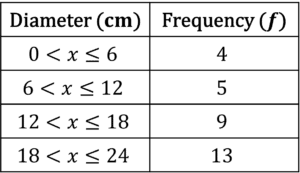Step 1: Add two new columns to the table, one called midpoint and one called midpoint $\times$ frequency.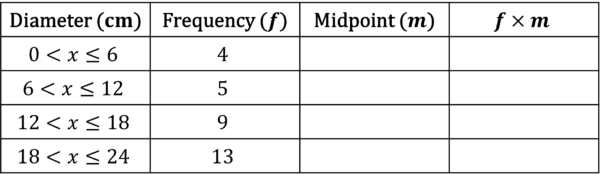Step 2: In the midpoint column, add the midpoint of each class.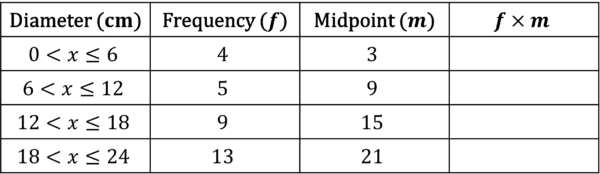Step 3: To calculate the values for the midpoint $\times$ frequency column, find the product of the frequency and midpoint for each row.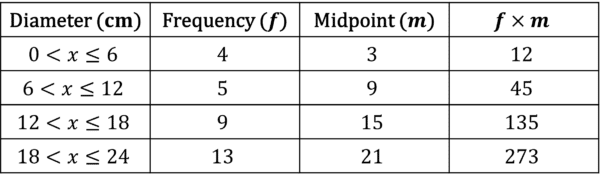Step 4: Find the totals of the frequency column, and the midpoint $\times$ frequency column.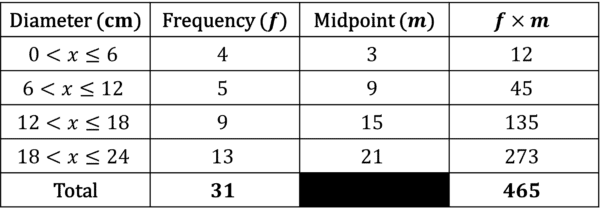Step 5: The estimate for the mean is the total of the midpoint $\times$ frequency column, divided by the total of the frequency column.

$465\div31=15$

So the estimate for the mean is $15\text{ cm}$.

Level 6-7GCSECambridge iGCSE

## Example 1: Median and Mode from a Grouped Frequency Table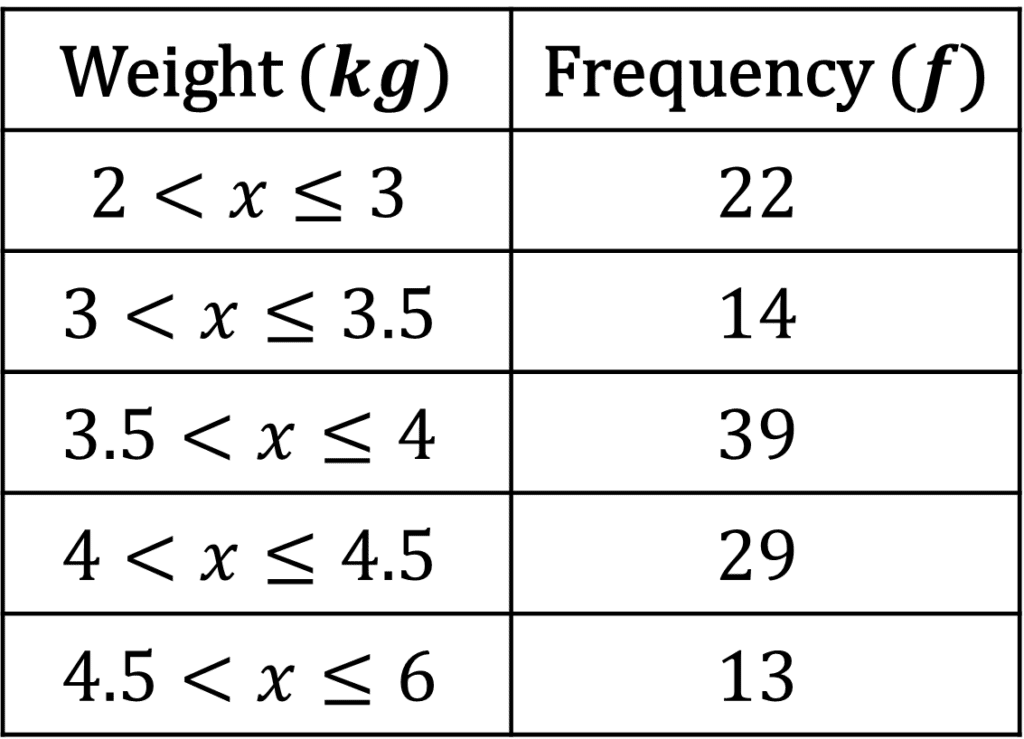The grouped frequency table on the right shows the weights of $117$ cats.

Find the modal class and the median class.

[3 marks]

The modal class is the class with the highest frequency, which in this case is $3.5.

The median class is the class containing the median value. Since there are $117$ cats, the median cat will be in the $59^{\text{th}}$ position.

Since $22+14=36<59$ and $22+14+39=75>59$, the median class must be $3.5.

Level 6-7GCSECambridge iGCSE

## Example 2: Estimating the Mean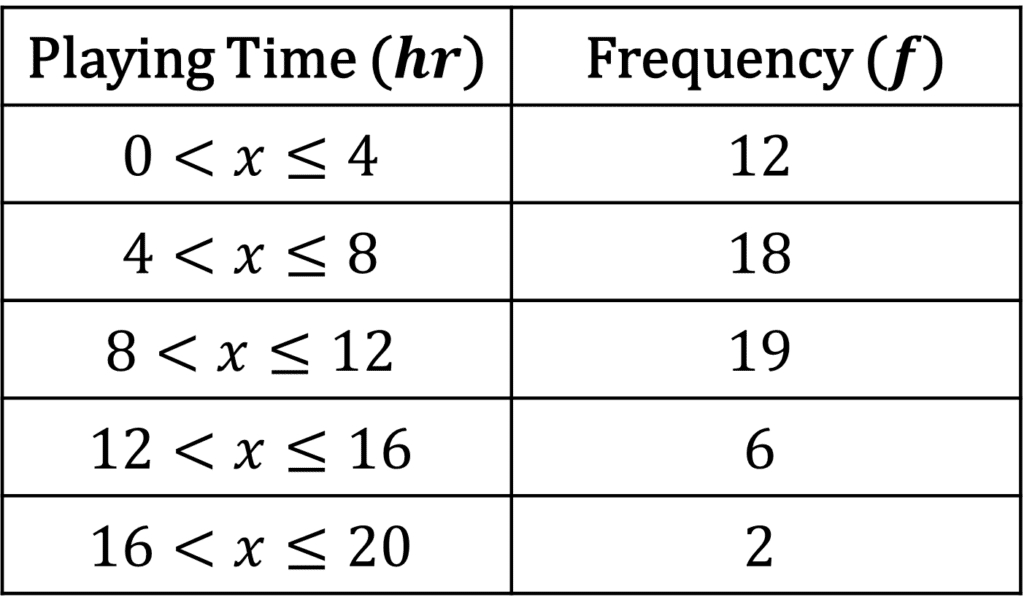Cindy asked multiple people how many hours they have spent playing video games in the past week. She collects her results in a table, pictured on the right.

Use the table to estimate the mean time spent playing video games.

[4 marks]

Step 1: Add two new columns to the table, one called midpoint and one called midpoint $\times$ frequency.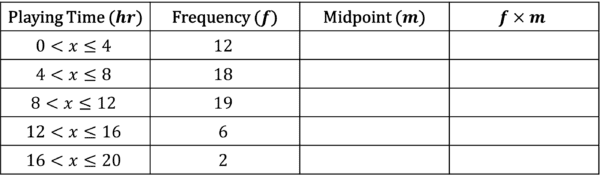Step 2: In the midpoint column, add the midpoint of each class.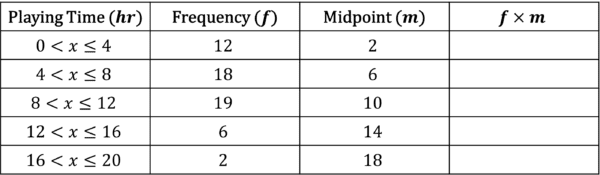Step 3: To calculate the values for the midpoint $\times$ frequency column, find the product of the frequency and midpoint for each row.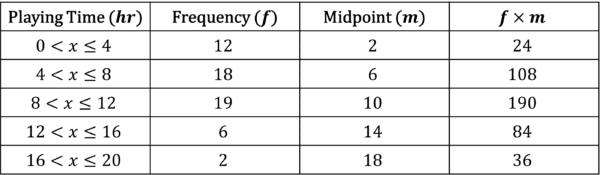Step 4: Find the totals of the frequency column, and the midpoint $\times$ frequency column.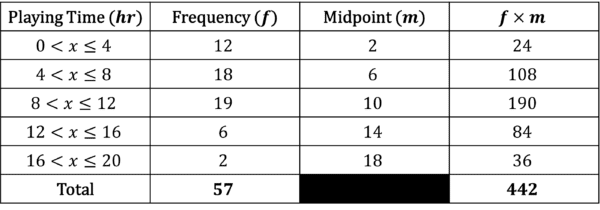Step 5: The estimate for the mean is the total of the midpoint $\times$ frequency column, divided by the total of the frequency column.

$442/57=7.75$ to two decimal places.

So the estimate for the mean is $7.75\text{ hrs}$.

Level 6-7GCSECambridge iGCSE

## Example 3: Estimating the MeanData on several cats is shown in the grouped frequency table on the right.

Calculate an estimate for the mean of the weight of the cats.

[4 marks]

Step 1: Add two new columns to the table, one called midpoint and one called midpoint $\times$ frequency.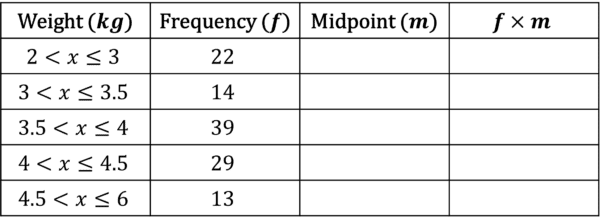Step 2: In the midpoint column, add the midpoint of each class.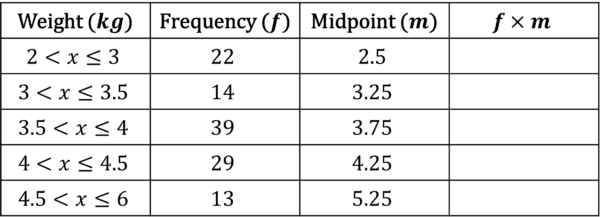Step 3: To calculate the values for the midpoint $\times$ frequency column, find the product of the frequency and midpoint for each row.Step 4: Find the totals of the frequency column, and the midpoint $\times$ frequency column.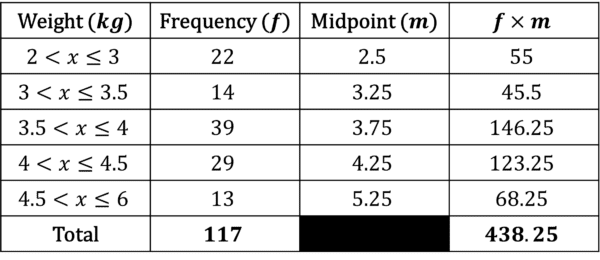Step 5: The estimate for the mean is the total of the midpoint $\times$ frequency column, divided by the total of the midpoint column.

$438.25/117=3.75$ to two decimal places.

So the estimate for the mean is $3.75\text{ kg}$.

Level 6-7GCSECambridge iGCSE

## Grouped Frequency Tables Example Questions

a) The completed frequency table is as follows: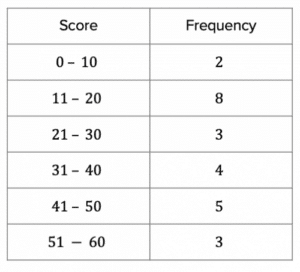b) The students who scored above $40$ are those in the $41-50$ and $51-60$ classes of the table, which is $5+3=8$ students.

$8\div25(\times100\%)=32\%$

a) The classes $2 and $3 are the classes of people who took longer than $2$ minutes.

Hence, $19+19=38$ people took longer than $2$ minutes.

b) $90\text{ s}$ is $1.5\text{ mins}$, so this is the top two classes: $0 and $1.

Hence, the number of people who completed the puzzle in $90\text{ s}$ or less is $8+22=30$.

$30\div100=\dfrac{3}{10}$

Step 1: Add two new columns to the table, one called midpoint and one called midpoint $\times$ frequency.Step 2: In the midpoint column, add the midpoint of each class.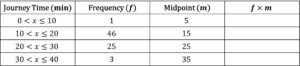Step 3: To calculate the values for the midpoint $\times$ frequency column, find the product of the frequency and midpoint for each row.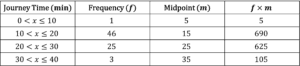Step 4: Find the totals of the frequency column, and the midpoint $\times$ frequency column.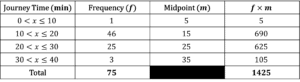Step 5: The estimate for the mean is the total of the midpoint $\times$ frequency column, divided by the total of the midpoint column.

$1425\div75=19\text{ min}$

Step 1: Add two new columns to the table, one called midpoint and one called midpoint $\times$ frequency.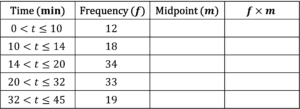Step 2: In the midpoint column, add the midpoint of each class.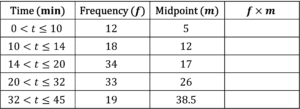Step 3: To calculate the values for the midpoint $\times$ frequency column, find the product of the frequency and midpoint for each row.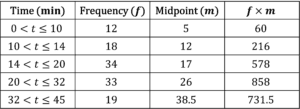Step 4: Find the totals of the frequency column, and the midpoint $\times$ frequency column.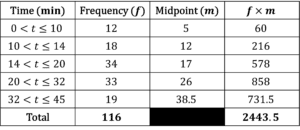Step 5: The estimate for the mean is the total of the midpoint $\times$ frequency column, divided by the total of the midpoint column.

$2443.5\div116=21\text{ min}$ to the nearest minute.

a) Step 1: Add two new columns to the table, one called midpoint and one called midpoint $\times$ frequency.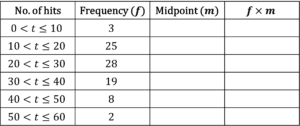Step 2: In the midpoint column, add the midpoint of each class.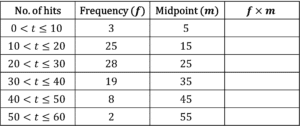Step 3: To calculate the values for the midpoint $\times$ frequency column, find the product of the frequency and midpoint for each row.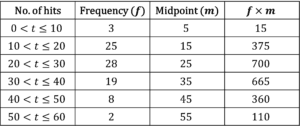Step 4: Find the totals of the frequency column, and the midpoint $\times$ frequency column.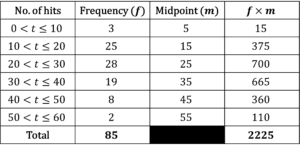Step 5: The estimate for the mean is the total of the midpoint $\times$ frequency column, divided by the total of the midpoint column.

$2225\div85=26$ hits to the nearest whole number.

b) Rhianna’s table looks like this: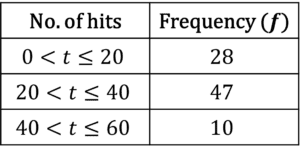Step 1: Add two new columns to the table, one called midpoint and one called midpoint $\times$ frequency.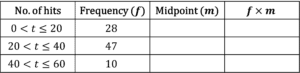Step 2: In the midpoint column, add the midpoint of each class.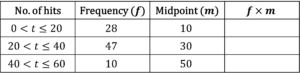Step 3: To calculate the values for the midpoint $\times$ frequency column, find the product of the frequency and midpoint for each row.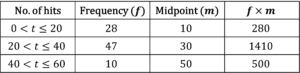Step 4: Find the totals of the frequency column, and the midpoint $\times$ frequency column.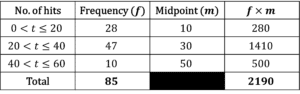Step 5: The estimate for the mean is the total of the midpoint $\times$ frequency column, divided by the total of the midpoint column.

$2190\div85=26$ hits to the nearest whole number.

## You May Also Like...### MME Learning Portal

Online exams, practice questions and revision videos for every GCSE level 9-1 topic! No fees, no trial period, just totally free access to the UK’s best GCSE maths revision platform.

£0.00